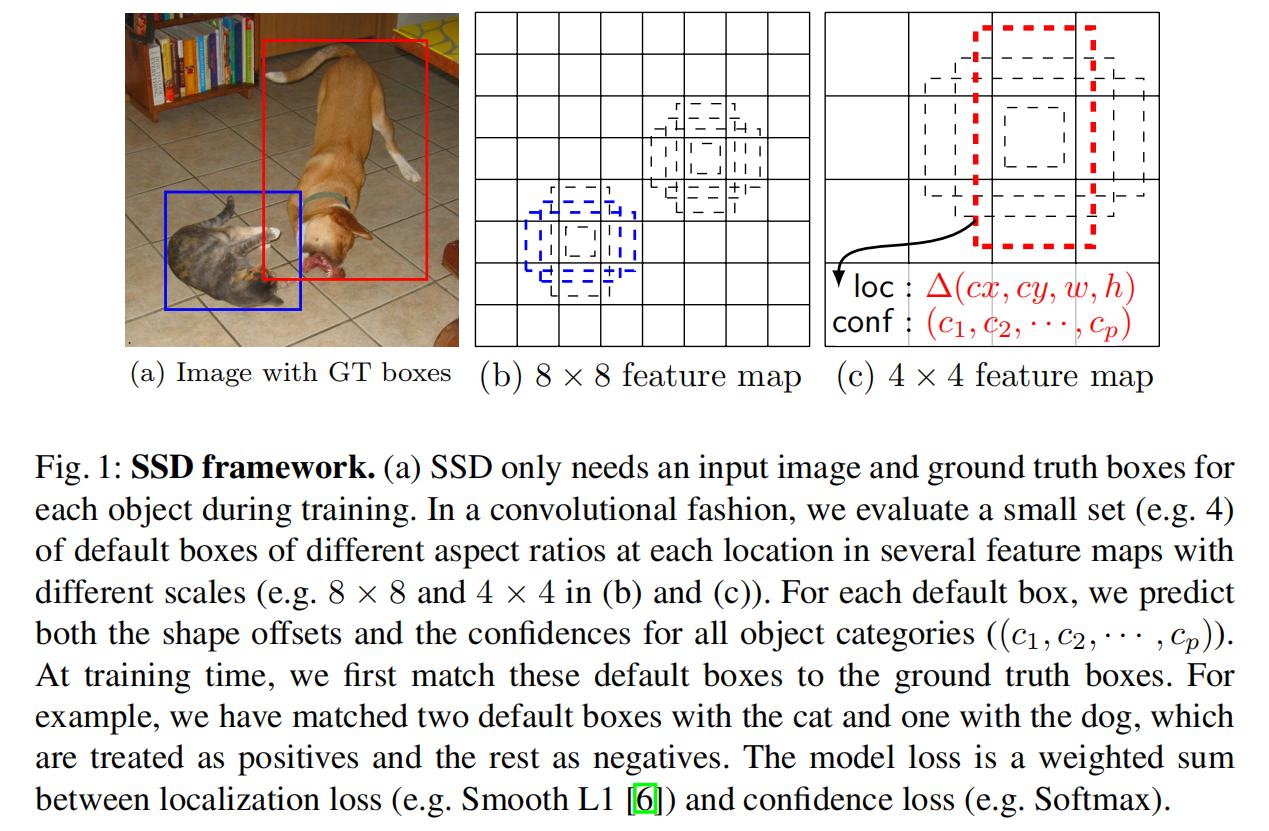# 先验框¶

SSD算法定义了多尺度、多长宽比的先验框（Prior Boxes，也称为默认框，Default Boxes），代替了目标预提取步骤，能够加速检测过程

## 尺度设置¶

s_{k} = s_{min} + \frac {s_{max} - s_{min}}{m-1}(k-1), k\in [1, m]

• $m$表示不同分辨率的特征图的个数
• $S_{min}=0.2, S_{max}=0.9$

## 长宽比设置¶

a_{r}\in \{1, 2, 3, \frac {1}{2}, \frac {1}{3}\}\\ w_{k}^{a} = s_{k}\sqrt {a_{r}} \\ h_{k}^{a} = s_{k} / \sqrt {a_{r}}

scale_{1} = s_{k} \ \ \ \ scale_{2} = \sqrt {s_{k} s_{k+1}}

## 中心坐标计算¶

(center_{x}, center_{y}) = (\frac {i+0.5}{\left | f_{k} \right |})

## 先验框个数¶

• Conv4_3$38\times 38\times 512$
• Conv7$19\times 19\times 1024$
• Conv8_2$10\times 10\times 512$
• Conv9_2$5\times 5\times 256$
• Conv10_2$3\times 3\times 256$
• Conv11_2$1\times 1\times 256$

Conv4_3/Conv10_2/Conv11_2中，每个cell使用了3种长宽比设置 - $(1, 2, \frac {1}{2})$，所以在这些特征图中每个cell包含了4个先验框（因为长宽比为1的先验框有2种尺度

Conv7/Conv8_2/Conv9_2中，每个cell使用了5种长宽比设置 - $(1, 2, 3, \frac{1}{2}, \frac{1}{3})$，所以在这些特征图中每个cell包含了6个先验框

(38\times 38 + 3\times 3+ 1\times 1)\times 4 + (19\times 19+ 10\times 10+ 5\times 5)\times 6=5816 + 2916 = 8732

## 先验框作用¶• 对分辨率较高的特征图使用小尺度的先验框
• 对分辨率较低的特征图使用大尺度的先验框

• 比较大的特征图能够用来检测相对较小的目标
• 比较小的特征图能够用来检测相对较大的目标

## 具体实现¶

• py/ssd/models/achors/prior_box.py

SSD先验框详解

{0.1, 0.2, 0.375, 0.55, 0.735, 0.9}

S_{k} = \left \lfloor S_{min} * 100 \right \rfloor + \left \lfloor \frac {\left \lfloor S_{max}*100 \right \rfloor - \left \lfloor S_{min}*100 \right \rfloor}{m-1} \right \rfloor (k-1)

{10, 20, 37, 54, 71, 88}

{0.1, 0.2, 0.37, 0.54, 0.71, 0.88}

{30, 60, 111, 162, 213, 264}